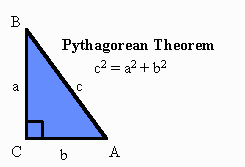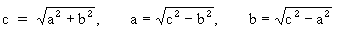# Pythagorean Theorem

The Pythagorean Theorem says that in any right triangle, the lengths of the hypotenuse and the other two sides are related by a simple formula.So, if you know the lengths of any two sides of a right triangle, you can use the Pythagorean Theorem to find the length of the third side:The Pythagorean Theorem is true for all right triangles. There are many ways, called proofs, to show that this is true.